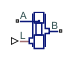# Annular Leakage (IL)

Models annular leakage between a circular tube and a round insert in an isothermal flow

Since R2020a

•Libraries:
Simscape / Fluids / Isothermal Liquid / Valves & Orifices / Orifices

## Description

The Annular Leakage (IL) block models annular leakage between a circular tube and a round insert in an isothermal liquid network. The insert can be located off-center from the tube and can have varying lengthwise overlap with the tube.Ports A and B correspond with the orifice inlet and outlet. The input ports L and E are optional physical signal ports that model variable overlap length (L) and variable eccentricity (E).

### The Mass Flow Rate Equation

The leakage mass flow rate is calculated from the pressure-flow rate equation

`$\stackrel{˙}{m}=\frac{\pi {\left(R-r\right)}^{3}\left(R+r\right)}{12v}\frac{\left({p}_{A}-{p}_{B}\right)}{l}\left[1+3{\epsilon }_{{}_{sat}}^{2}\frac{R}{\left(R+r\right)}+\frac{3}{8}{\epsilon }^{4}\frac{\left(R-r\right)}{\left(R+r\right)}\right],$`

where:

• R is the annulus outer radius.

• r is the annulus inner radius.

• pA is the pressure at port A.

• pB is the pressure at port B.

• ν is the fluid kinematic viscosity.

• l is the overlap length.

• ε is the eccentricity ratio, $\epsilon =\frac{e}{R-r}$, where e is the eccentricity, which can be defined as a physical signal or constant value.

• εsat is the saturated eccentricity ratio, which is ε for constant orifices, or the physical signal connected to port L for variable orifices. The eccentricity ratio is always between 0 and 1.

When modeling a variable overlap length, the user-defined minimum overlap length is used if the physical signal falls below the value of the Minimum overlap length parameter.

### Assumptions and Limitations

The pressure-flow equation is valid only for fully-developed, laminar flows. The flow Reynolds number can be determined using $\mathrm{Re}=\frac{\stackrel{˙}{m}{D}_{h}}{\mu \pi \left({R}^{2}-{r}^{2}\right)}$ or by checking the simulation log in the Results Explorer or the Simulation Data Inspector. For more information, see Data Logging.

## Ports

### Conserving

expand all

Entry or exit port of the liquid to or from the orifice.

Entry or exit port of the liquid to or from the orifice.

### Input

expand all

Optional physical signal port that provides the variable overlap length between the sleeve and annular insert.

#### Dependencies

To expose this port, set Overlap length specification to `Variable`.

Optional physical signal port that provides variable eccentricity of the annular insert to the sleeve.

#### Dependencies

To expose this port, set Eccentricity specification to `Variable`.

## Parameters

expand all

Whether the length-wise sleeve-insert overlap is constant or variable.

Length-wise overlap between the insert and tube.

#### Dependencies

To enable this parameter, set Overlap length specification to `Constant`.

Lower limit to the length-wise overlap between the insert and tube. Any length shorter than this value will be set to the specified minimum.

#### Dependencies

To enable this parameter, set Overlap length specification to `Variable`.

Whether the orifice eccentricity changes or remains at a set value.

The distance between the center of the orifice and the center of the insert.

#### Dependencies

To enable this parameter, set Eccentricity specification to `Constant`.